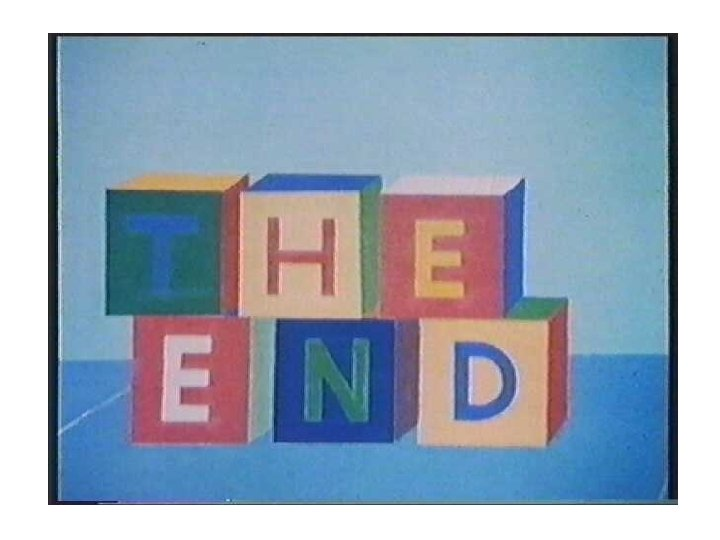# Unit 6 Balancing Chemical Equations Chemical Reactions Stoichiometry

• Slides: 46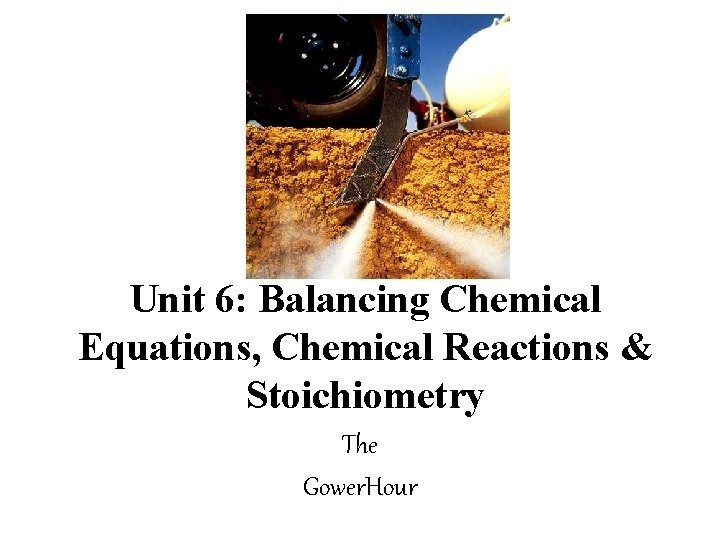Unit 6: Balancing Chemical Equations, Chemical Reactions & Stoichiometry The Gower. Hour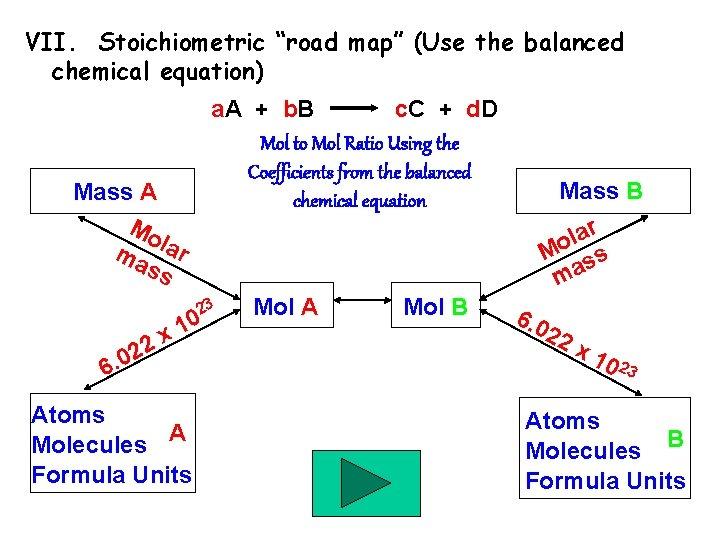VII. Stoichiometric “road map” (Use the balanced chemical equation) a. A + b. B c. C + d. D Mol to Mol Ratio Using the Coefficients from the balanced chemical equation Mass A Mo ma lar ss 23 2 2 0 6. 0 1 x Atoms A Molecules Formula Units Mol A Mol B Mass B r a l Mo ss ma 6. 0 22 x 1 0 23 Atoms B Molecules Formula Units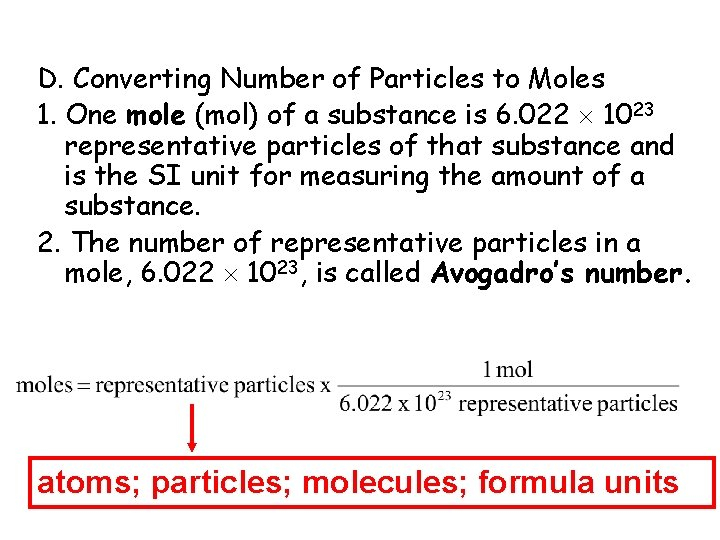10. 1 D. Converting Number of Particles to Moles 1. One mole (mol) of a substance is 6. 022 1023 representative particles of that substance and is the SI unit for measuring the amount of a substance. 2. The number of representative particles in a mole, 6. 022 1023, is called Avogadro’s number. atoms; particles; molecules; formula units3. Magnesium is a light metal used in the manufacture of aircraft, automobile wheels, tools, and garden furniture. How many moles of magnesium is 1. 25 x 1023 atoms of magnesium? 1 mol Mg atoms = 6. 022 x 1023 atoms Mg 1 mol Mg atoms 1. 25 x 1023 atoms Mg X 6. 022 x 1023 atoms Mg = 0. 208 mol Mg = 2. 08 x 10 -1 mol Mg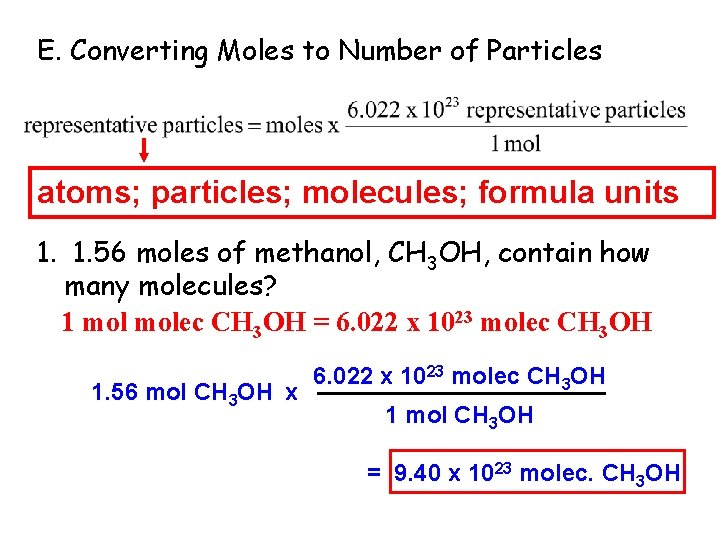10. 1 E. Converting Moles to Number of Particles atoms; particles; molecules; formula units 1. 1. 56 moles of methanol, CH 3 OH, contain how many molecules? 1 molec CH 3 OH = 6. 022 x 1023 molec CH 3 OH 1. 56 mol CH 3 OH x 6. 022 x 1023 molec CH 3 OH 1 mol CH 3 OH = 9. 40 x 1023 molec. CH 3 OHCalculating Molecular Mass Calculate the molecular mass of magnesium carbonate, Mg. CO 3. 24. 31 g + 12. 01 g + 3(16. 00 g) = 84. 32 g/mol2. Aluminum satellite dishes are resistant to corrosion because the aluminum reacts with oxygen in the air to form a coating of aluminum oxide (Al 2 O 3). This tough, resistant coating prevents any further corrosion. What is the mass of 9. 45 mol of aluminum oxide? 1 mol Al 2 O 3 = 101. 96 g Al 2 O 3 9. 45 mol Al 2 O 3 x 101. 96 g Al 2 O 3 1 mol Al 2 O 3 = 964 g Al 2 O 34. When iron is exposed to air, it corrodes to form red-brown rust. Rust is iron(III) oxide (Fe 2 O 3). How many moles of iron(III) oxide are contained in 92. 2 g of pure Fe 2 O 3? 1 mol Fe 2 O 3 = 159. 70 g Fe 2 O 3 92. 2 g Fe 2 O 3 x 1 mol Fe 2 O 3 159. 70 g Fe 2 O 3 = 0. 577 mol Fe 2 O 3 = 5. 77 x 10 -1 mol Fe 2 O 3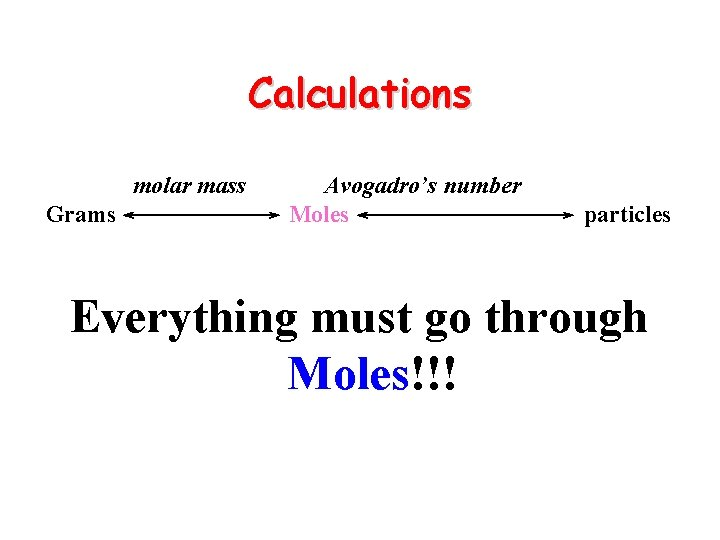Calculations molar mass Grams Avogadro’s number Moles particles Everything must go through Moles!!!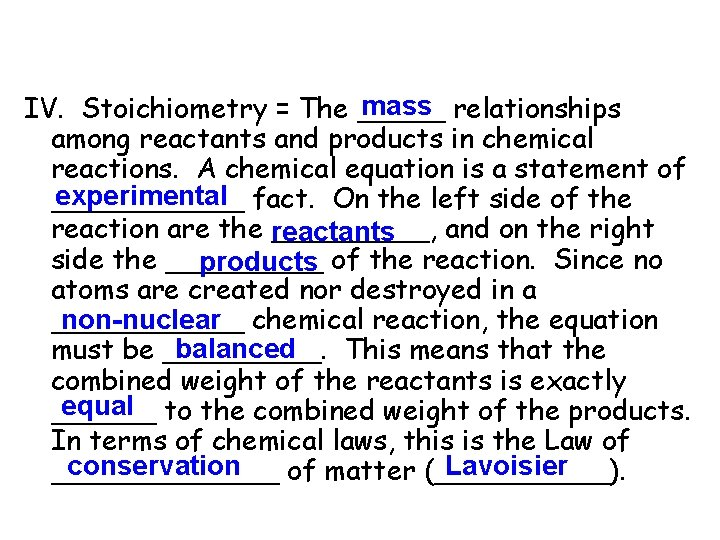mass relationships IV. Stoichiometry = The _____ among reactants and products in chemical reactions. A chemical equation is a statement of experimental fact. On the left side of the ______ reaction are the reactants _____, and on the right side the _____ products of the reaction. Since no atoms are created nor destroyed in a ______ non-nuclear chemical reaction, the equation balanced must be _____. This means that the combined weight of the reactants is exactly equal to the combined weight of the products. ______ In terms of chemical laws, this is the Law of conservation Lavoisier _______ of matter (_____).V. Limiting and Excess Reagents A. In a chemical reaction, an insufficient quantity of any of the reactants will limit the amount of product that forms. limiting reagent is the reagent that B. The _______ determines the amount of product that can be formed by a reaction. C. In the reaction of nitrogen and hydrogen, hydrogen is the limiting reagent. Nitrogen is the reagent that is not completely used up in the reaction. The reagent that is not used up reagent is called the excess ______.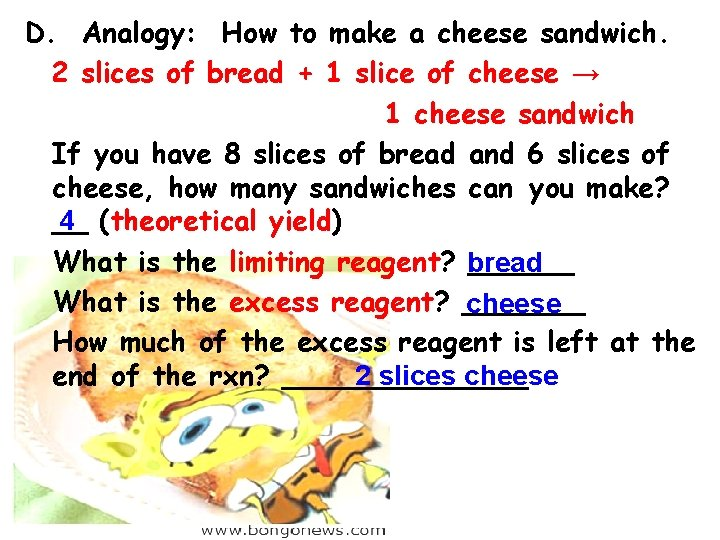D. Analogy: How to make a cheese sandwich. 2 slices of bread + 1 slice of cheese → 1 cheese sandwich If you have 8 slices of bread and 6 slices of cheese, how many sandwiches can you make? 4 (theoretical yield) __ What is the limiting reagent? bread ______ What is the excess reagent? _______ cheese How much of the excess reagent is left at the 2 slices cheese end of the rxn? _______VI. Percent Yield A. The percent yield is a measure of the efficiency of a reaction carried out in the _____ laboratory. B. A batting average is actually a percent yield.theoretical C. The ______ yield is the maximum amount of product that could be formed from given amounts of reactants. D. In contrast, the amount of product that actually forms when the reaction is carried laboratory is called the actual out in the _____ yield. E. The percent yield is the ratio of the actual yield to theoretical yield expressed as a percent.VII. Stoichiometric “road map” (Use the balanced chemical equation) a. A + b. B c. C + d. D Mol to Mol Ratio Using the Coefficients from the balanced chemical equation Mass A Mo ma lar ss 23 2 2 0 6. 0 1 x Atoms A Molecules Formula Units Mol A Mol B Mass B r a l Mo ss ma 6. 0 22 x 1 0 23 Atoms B Molecules Formula UnitsExamples: A. Calcium reacts with oxygen to form calcium oxide. If you are given 80. 0 g of calcium, (1) Calculate theoretical yield of calcium oxide. (2) How many moles of your excess reagent (ER) are used? LR 2 Ca + O 2 2 Ca. O ER 1 mol Ca 2 mol Ca. O 56. 08 g Ca. O (1) 80. 0 g Ca x x x 40. 08 g Ca 2 mol Ca 1 mol Ca. O LR = 112 g Ca. O Theoretical Yield ER 1 mol Ca 1 mol O 2 (2) 80. 0 g Ca x x 40. 08 g Ca 2 mol Ca LR = 0. 998 mol O 2 ER used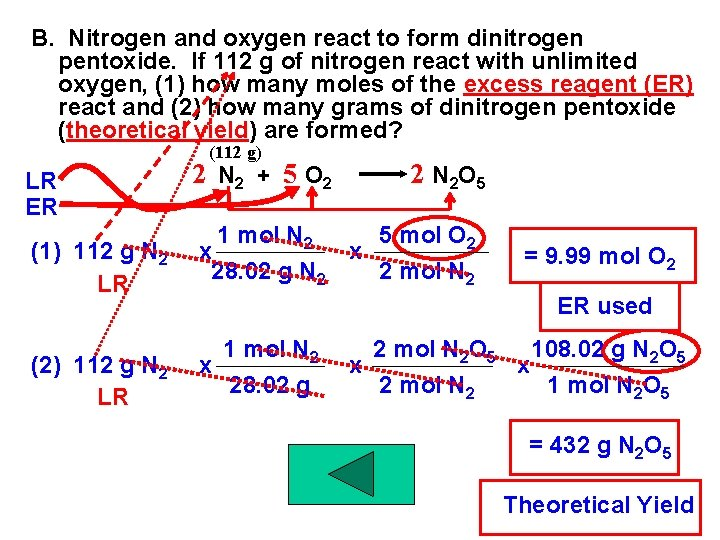B. Nitrogen and oxygen react to form dinitrogen pentoxide. If 112 g of nitrogen react with unlimited oxygen, (1) how many moles of the excess reagent (ER) react and (2) how many grams of dinitrogen pentoxide (theoretical yield) are formed? (112 g) LR ER 2 N 2 + 5 O 2 2 N 2 O 5 (1) 112 g N 2 LR 1 mol N 2 5 mol O 2 x x 28. 02 g N 2 2 mol N 2 (2) 112 g N 2 LR 1 mol N 2 x 28. 02 g = 9. 99 mol O 2 ER used 2 mol N 2 O 5 108. 02 g N 2 O 5 x x 2 mol N 2 1 mol N 2 O 5 = 432 g N 2 O 5 Theoretical Yield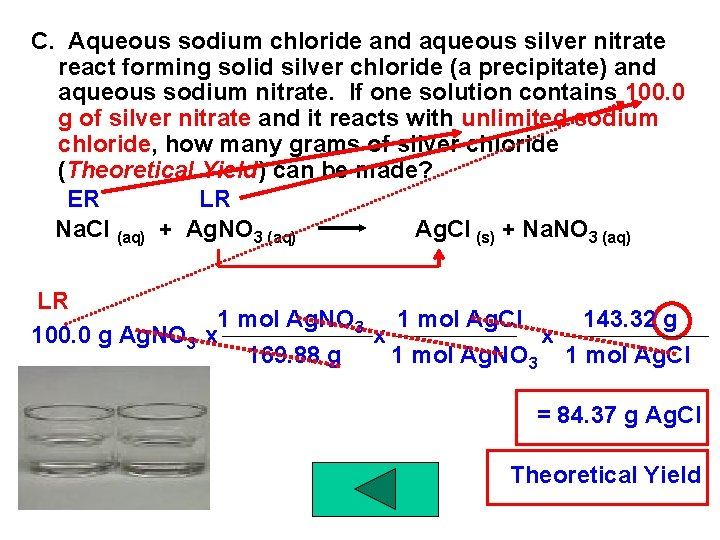C. Aqueous sodium chloride and aqueous silver nitrate react forming solid silver chloride (a precipitate) and aqueous sodium nitrate. If one solution contains 100. 0 g of silver nitrate and it reacts with unlimited sodium chloride, how many grams of silver chloride (Theoretical Yield) can be made? ER LR Na. Cl (aq) + Ag. NO 3 (aq) Ag. Cl (s) + Na. NO 3 (aq) LR 1 mol Ag. NO 3 1 mol Ag. Cl 143. 32 g 100. 0 g Ag. NO 3 x x x 169. 88 g 1 mol Ag. NO 3 1 mol Ag. Cl = 84. 37 g Ag. Cl Theoretical Yield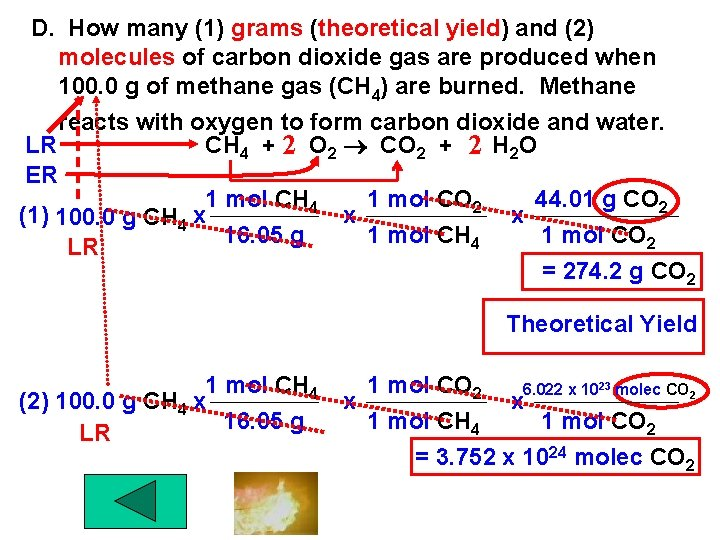D. How many (1) grams (theoretical yield) and (2) molecules of carbon dioxide gas are produced when 100. 0 g of methane gas (CH 4) are burned. Methane reacts with oxygen to form carbon dioxide and water. LR CH 4 + 2 O 2 CO 2 + 2 H 2 O ER 1 mol CH 4 1 mol CO 2 44. 01 g CO 2 (1) 100. 0 g CH 4 x x x 16. 05 g 1 mol CH 4 1 mol CO 2 LR = 274. 2 g CO 2 Theoretical Yield 1 mol CH 4 (2) 100. 0 g CH 4 x 16. 05 g LR 1 mol CO 2 6. 022 x 1023 molec CO 2 x x 1 mol CH 4 1 mol CO 2 = 3. 752 x 1024 molec CO 2E. Nitrogen reacts with hydrogen gas forming ammonia (nitrogen trihydride). How many (1) grams of hydrogen and (2) moles of nitrogen are required to form 1. 00 pound of ammonia? N 2 + 3 H 2 2 NH 3 (1) 1. 00 lb NH 3 453. 6 g NH 3 1 mol NH 3 3 mol H 2 2. 02 g H 2 x x 1 lb 17. 04 g 2 mol NH 3 1 mol H 2 = 80. 7 g H 2 (2) 1. 00 lb NH 3 453. 6 g NH 3 1 mol N 2 x x x = 1 lb 17. 04 g 2 mol NH 3 13. 3 mol N 2Review Question: Nitrogen and hydrogen gas combine to form hydrazine (dinitrogen tetrahydride) which is used for rocket fuel. How many grams of nitrogen gas are needed to form 155 g of hydrazine? N 2 + 2 H 2 N 2 H 4 155 g N 2 H 4 1 mol N 2 28. 02 g N 2 x x x 32. 06 g 1 mol N 2 H 4 1 mol N 2 = 135 g N 2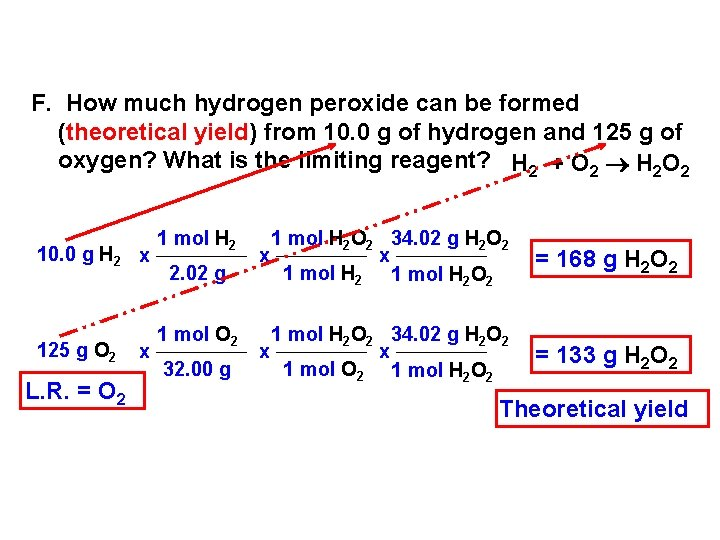F. How much hydrogen peroxide can be formed (theoretical yield) from 10. 0 g of hydrogen and 125 g of oxygen? What is the limiting reagent? H 2 + O 2 H 2 O 2 10. 0 g H 2 x 125 g O 2 L. R. = O 2 x 1 mol H 2 2. 02 g 1 mol O 2 32. 00 g 1 mol H 2 O 2 34. 02 g H 2 O 2 x x 1 mol H 2 O 2 = 168 g H 2 O 2 1 mol H 2 O 2 34. 02 g H 2 O 2 x x 1 mol O 2 1 mol H 2 O 2 = 133 g H 2 O 2 Theoretical yieldG. Determine theoretical yield of water that can be formed from 10. 0 g of hydrogen and 100. 0 g of oxygen. What is the limiting reagent? How much excess ER reagent is remaining? 2 H +O 2 H O 2 10. 0 g H 2 x L. R. = H 2 100. 0 g O 2 x 10. 0 g H 2 x 1 mol H 2 2. 02 g 1 mol O 2 32. 00 g 1 mol H 2 2. 02 g x x 1 mol H 2 O 1 mol H 2 2 mol H 2 O x 1 mol O 2 2 mol H 2 x x 18. 02 g H 2 O 1 mol H 2 O x 32. 00 g O 2 1 mol O 2 2 2 = 89. 2 g H 2 O Theoretical yield = 112. 6 g H 2 O = 79. 2 g O 2 ER used 100. 0 g O 2 79. 2 g O 2 = 20. 8 g O 2 remainingH. How many grams of aluminum oxide can be formed (theoretical yield) from 100. 0 g of aluminum and 100. 0 g of oxygen gas? How much excess reagent is remaining? 4 Al + 3 O 2 2 Al 2 O 3 100. 0 g Al x L. R. = Al 100. 0 g O 2 x 100. 0 g Al x 1 mol Al 26. 98 g 2 mol Al 2 O 3 101. 96 g x x 4 mol Al 1 mol Al 2 O 3 = 189. 0 g Al 2 O 3 Theoretical yield 1 mol O 2 32. 00 g 1 mol Al 26. 98 g x 2 mol Al 2 O 3 x 3 mol O 2 4 mol Al x 101. 96 g 1 mol Al 2 O 3 x 32. 00 g O 2 1 mol O 2 = 212. 4 g Al 2 O 3 = 88. 95 g O 2 100. 0 g O 2 - 88. 95 g O 2 = 11. 05 g O 2 RemainingI. A student does a lab in which she makes 2. 17 g of Lead (II) nitrate. From her limiting reagent she calculated that she should have made 2. 55 g. Determine: (1) theoretical yield, (2) actual yield, (3) % yield (4) absolute and relative error. (1) 2. 55 g Pb(NO 3)2 (2) 2. 17 g Pb(NO 3)2 (4) Ea = 2. 55 g – 2. 17 g = 0. 38 g Note: % yield + Er = 100 %J. A sample of 100. 0 g of hydrazine (N 2 H 4) burns in 280. 0 g of oxygen gas and 97. 2 g of water are formed. Calculate the % yield of water. (Nitrogen dioxide is also produced. ) Calculate the % error (relative error). N 2 H 4 + 3 O 2 2 H 2 O + 2 NO 2 1 mol N 2 H 4 2 mol H 2 O 18. 02 g 100. 0 g N 2 H 4 x x x 32. 06 g 1 mol N 2 H 4 1 mol H 2 O 280. 0 g O 2 x LR 1 mol O 2 32. 00 g x 2 mol H 2 O 3 mol O 2 x 18. 02 g 1 mol H 2 O = 112. 4 g H 2 O = 105. 1 g H 2 O Theo. yield % error = 100% - 92. 5% = 7. 5%K. In lab a student mixes a solution that contains 1. 70 g silver nitrate and a solution that contains 0. 832 g calcium chloride. Aqueous calcium nitrate and solid silver chloride are produced. After washing, filtering, and drying the precipitate, he finds that he has obtained 1. 25 g of silver chloride. What is the % yield? 2 Ag. NO 3 + Ca. Cl 2 Ca(NO 3)2 + 2 Ag. Cl (s) 1 mol Ag. NO 3 2 mol Ag. Cl 143. 32 g 1. 70 g Ag. NO 3 x x x 169. 88 g 2 mol Ag. NO 3 1 mol Ag. Cl = 1. 43 g Ag. Cl 1 mol Ca. Cl 2 2 mol Ag. Cl 143. 32 g 0. 832 g Ca. Cl 2 x x x 110. 98 g 1 mol Ca. Cl 2 1 mol Ag. Cl = 2. 15 g Ag. Cl Theo. yield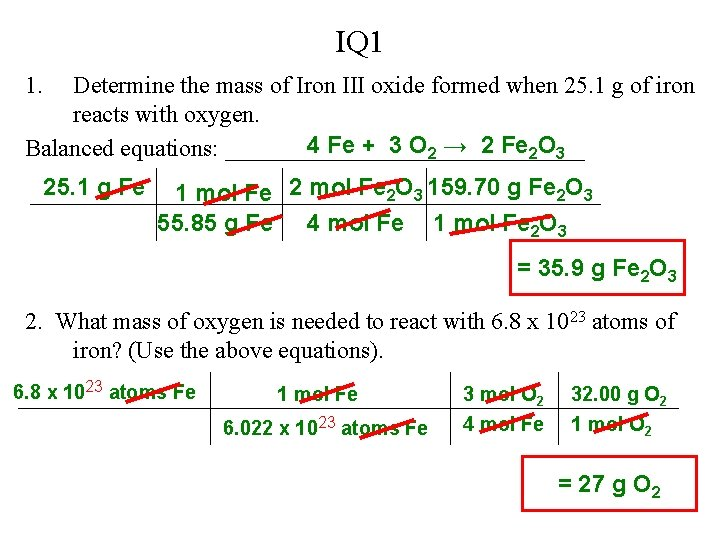IQ 1 1. Determine the mass of Iron III oxide formed when 25. 1 g of iron reacts with oxygen. 4 Fe + 3 O 2 → 2 Fe 2 O 3 Balanced equations: _______________ 25. 1 g Fe 1 mol Fe 2 O 3 159. 70 g Fe 2 O 3 55. 85 g Fe 4 mol Fe 1 mol Fe 2 O 3 = 35. 9 g Fe 2 O 3 2. What mass of oxygen is needed to react with 6. 8 x 1023 atoms of iron? (Use the above equations). 6. 8 x 1023 atoms Fe 1 mol Fe 6. 022 x 1023 atoms Fe 3 mol O 2 4 mol Fe 32. 00 g O 2 1 mol O 2 = 27 g O 2IQ 2 Determine the (a) limiting reagent and (b) theoretical yield of iron when 82. 3 g of iron (III) oxide reacts with 79. 3 g of carbon monoxide. (c) Grams of excess reagent remaining. Fe 2 O 3 + 3 CO → 2 Fe + 3 CO 2 Balance equ. : _________________ (a) 82. 3 g Fe 2 O 3 1 mol Fe O 3 mol CO 28. 01 g CO 2 3 159. 70 g Fe 2 O 3 1 mol CO Fe 2 O 3 – Limiting Reagent 43. 3 g CO needed (b) 82. 3 g Fe 2 O 3 1 mol Fe 2 O 3 2 mol Fe 55. 85 g Fe 159. 70 g Fe 2 O 3 1 mol Fe (c) Grams excess reagent remaining? Excess = grams @ start – grams reacted Excess = 79. 3 g CO – 43. 3 g CO = 36. 0 g CO 57. 6 g Fe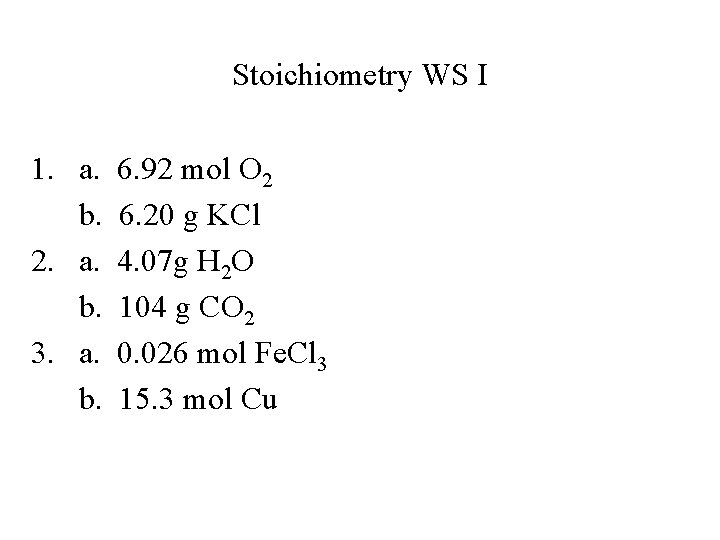Stoichiometry WS I 1. a. b. 2. a. b. 3. a. b. 6. 92 mol O 2 6. 20 g KCl 4. 07 g H 2 O 104 g CO 2 0. 026 mol Fe. Cl 3 15. 3 mol Cu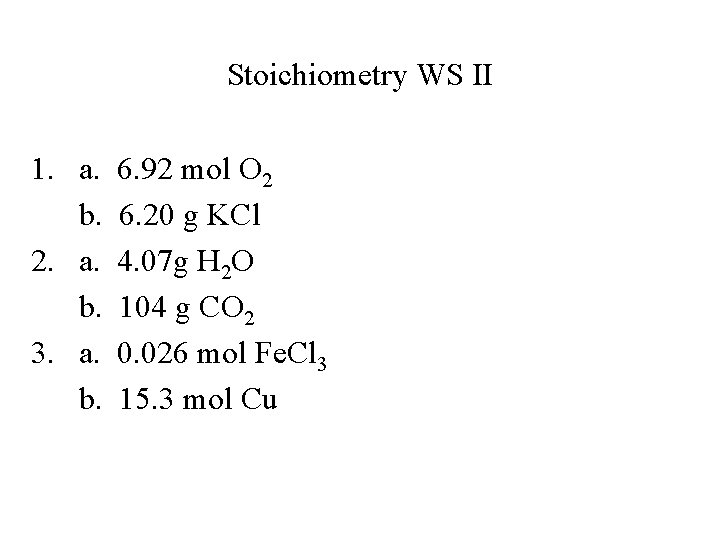Stoichiometry WS II 1. a. b. 2. a. b. 3. a. b. 6. 92 mol O 2 6. 20 g KCl 4. 07 g H 2 O 104 g CO 2 0. 026 mol Fe. Cl 3 15. 3 mol CuSample Test Part A 1. (1) Syn (2) Decomp (3) DD (4) SD (5) Combustion 2. a. 1, 4, 3, 4 b. 1, 1, 1, 1 c. 6, 1, 2, 3 d. 2, 3, 1, 3 1. a. 4, 3, 2 (Syn) b. 2, 2, 2, 1 (SD) c. 2, 7, 4, 6 (Comb) d. 1, 2, 1, 2 (DD) e. 2, 1, 1 (Decomp) 4. a. 1, 1, 2 Ag. Br, 1 Ca. SO 4 b. 2, 1 H 2, 1 Br 2 c. 1, 5, 3 CO 2, 4 H 2 O d. 2, 3, 1 Al 2 S 3 e. 2, 1 N 2, 3 H 2Sample Test Part A 5. a. 2, 3, 1 Al 2(SO 4)3 Cu b. 1, 2, 1 Ca(OH)2, 1 H 2 c. 1, 2, 1 Fe(OH)2, 1 H 2 d. 1, 2, 1 Zn. Cl 2, 1 H 2 e. NR f. 1, 2, 1 Cu(NO 3)2, 2 Ag 6. HOFBr. INCl 7. Speeds up rxn w/o being used up in the rxn. 8. C, CO 2, H 2 O 9. See Metal activities series.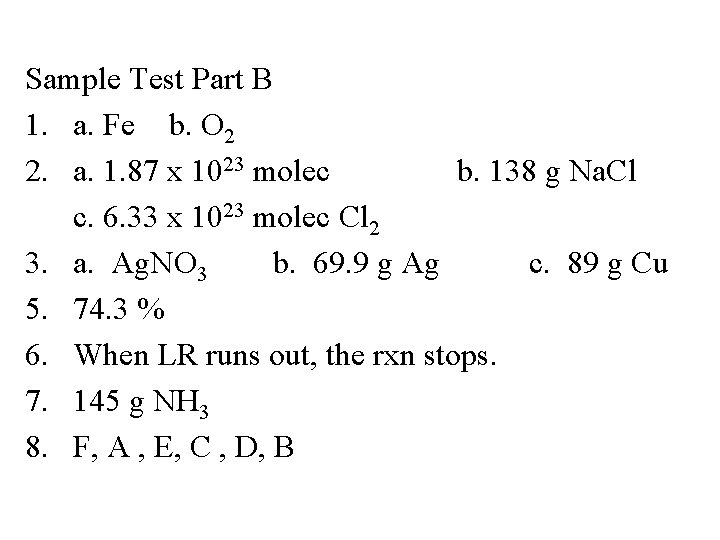Sample Test Part B 1. a. Fe b. O 2 2. a. 1. 87 x 1023 molec b. 138 g Na. Cl c. 6. 33 x 1023 molec Cl 2 3. a. Ag. NO 3 b. 69. 9 g Ag c. 89 g Cu 5. 74. 3 % 6. When LR runs out, the rxn stops. 7. 145 g NH 3 8. F, A , E, C , D, B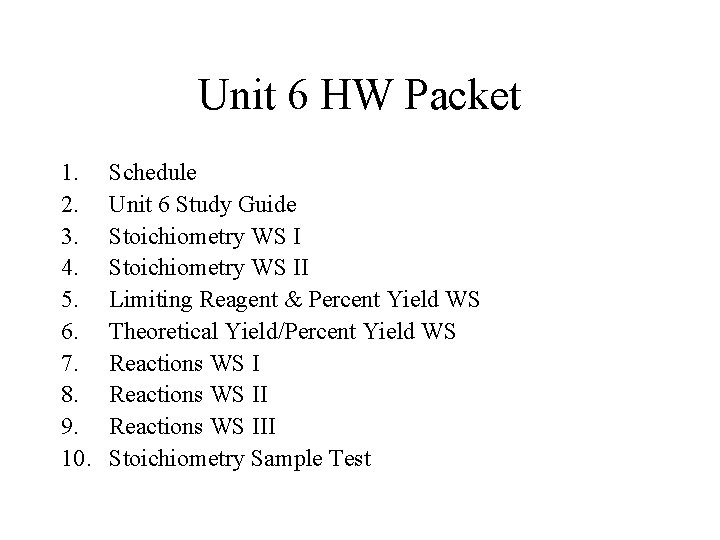Unit 6 HW Packet 1. 2. 3. 4. 5. 6. 7. 8. 9. 10. Schedule Unit 6 Study Guide Stoichiometry WS II Limiting Reagent & Percent Yield WS Theoretical Yield/Percent Yield WS Reactions WS III Stoichiometry Sample TestChemistry WS 1. a. b. 2. a. b. 3. a. b. 6. 92 mol O 2 6. 20 g KCl 4. 07 g H 2 O 104 g CO 2 0. 026 mol Fe. Cl 3 15. 3 mol CuStoichiometry WS 1. 2. 3. 4. 5. 6. 1. 81 x 1023 molecules H 2 7. 3 g H 2 3. 09 mol Al 2(SO 4)3 22 g Al 6. 7 x 1022 atoms Al 8. 1 g H 2Limiting Reagent & Percent Yield WS 1. a. b. c. 2. a. b. 3. a. b. L. R. = HCl L. R. = O 2 L. R. = Cl 2 L. R. = HCl; 0. 26 mol H 2 produced 0. 26 mol Zn used; 0. 04 mol Zn excess L. R. = HCl; 0. 12 mol Al. Cl 3 produced 0. 12 mol Al used; 0. 03 mol Al excessLimiting Reagent & Percent Yield 4. 87. 9 % 5. 75. 2 % Theoretical Yield/Percent Yield 4. 54. 2 % 5. Actual yield = 1660 g NH 3 6. 37. 0 % 7. Actual yield = 58. 7 g Mg. OReaction Types 1. DD: Al 2(SO 4)3 + Ca 3(PO 4)2 2 Al. PO 4 + 3 Ca. SO 4 2. DD: Mg. Cl 2 + 2 Ag. NO 3 Mg(NO 3)2 + 2 Ag. Cl 4. Syn: 2 H 2 + O 2 2 H 2 O 5. SD: Zn + Cu(NO 3)2 Zn(NO 3)2 + Cu 7. DD: Cu. O + H 2 SO 4 Cu. SO 4 + H 2 O 9. SD: 2 Na. I + Cl 2 2 Na. Cl + I 2 10. Decomp: Cu(OH)2 Cu. O + H 2 O 12. SD: K + H 2 O KOH + H 2Sample Test Part A 1. (1) Syn (2) Decomp (3) DD (4) SD (5) Combustion 2. a. 1, 4, 3, 4 b. 1, 1, 1, 1 c. 6, 1, 2, 3 d. 2, 3, 1, 3 1. a. 4, 3, 2 (Syn) b. 2, 2, 2, 1 (SD) c. 2, 7, 4, 6 (Comb) d. 1, 2, 1, 2 (DD) e. 2, 1, 1 (Decomp) 4. a. 1, 1, 2 Ag. Br, 1 Ca. SO 4 b. 2, 1 H 2, 1 Br 2 c. 1, 5, 3 CO 2, 4 H 2 O d. 2, 3, 1 Al 2 S 3 e. 2, 1 N 2, 3 H 2Sample Test Part A 5. a. 2, 3, 1 Al 2(SO 4)3 Cu b. 1, 2, 1 Ca(OH)2, 1 H 2 c. 1, 2, 1 Fe(OH)2, 1 H 2 d. 1, 2, 1 Zn. Cl 2, 1 H 2 e. NR f. 1, 2, 1 Cu(NO 3)2, 2 Ag 6. HOFBr. INCl 7. Speeds up rxn w/o being used up in the rxn. 8. C, CO 2, H 2 O 9. See Metal activities series.Sample Test Part B 1. a. Fe b. O 2 2. a. 1. 87 x 1023 molec b. 138 g Na. Cl c. 6. 33 x 1023 molec Cl 2 3. a. Ag. NO 3 b. 69. 9 g Ag c. 89 g Cu 5. 74. 3 % 6. When LR runs out, the rxn stops. 7. 145 g NH 3 8. F, A , E, C , D, BStoichiometry Review WS 1. a. N 2 O 5 + H 2 O 2 HNO 3 b. 111. 8 g HNO 3 c. 15. 7 g H 2 O 2. a. 4 Al + 3 Pb. O 2 2 Al 2 O 3 + 3 Pb b. 2. 113 g Al 3. a. LR = H 2 S b. 8. 257 g (NH 4)2 S c. 2. 709 g NH 3 4. a. Yes; SD; Cl 2 + 2 KBr 2 KCl + Br 2 b. LR = Cl 2 c. 1. 93 g Br 2 d. 0. 335 g KBr 5. a. LR = As 2 O 3 b. 79. 3 % 6. a. 6, 6, 1, 6 b. 6. 32 x 103 g O 2 7. a. 71 % b. 26 metric tonsUnit 6 HW Packet 1. 2. 3. 4. 5. 6. 7. 8. 9. 10. Schedule Unit 6 Study Guide Stoichiometry WS II Limiting Reagent & Percent Yield WS Theoretical Yield/Percent Yield WS Reactions WS III Stoichiometry Sample Test